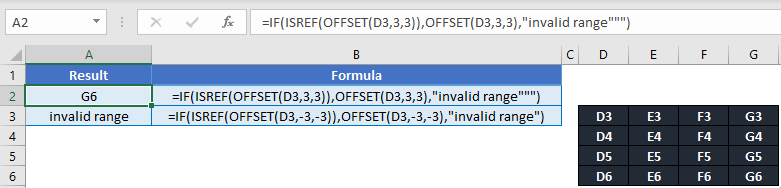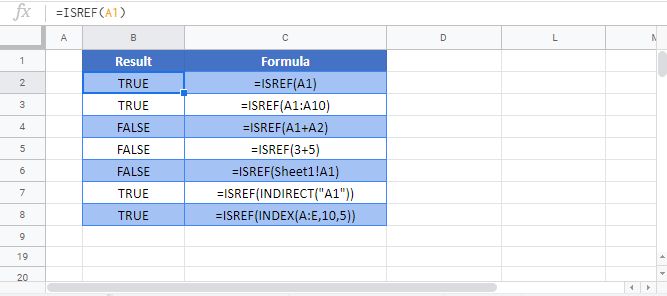# ISREF Function Examples – Excel & Google Sheets

This tutorial demonstrates how to use the Excel ISREF Function in Excel to test if a cell contains a reference.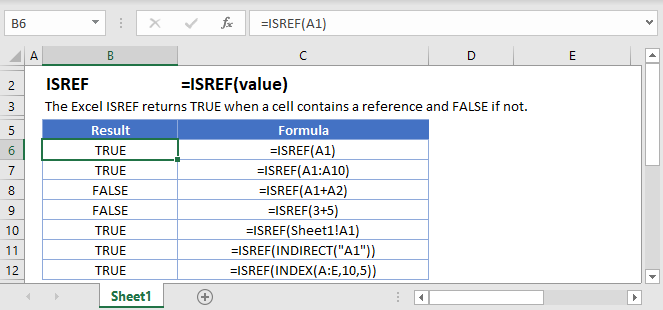## ISREF Function Description:

The ISREF Function Test if cell value is a reference. Returns TRUE or FALSE.

To use the ISREF Excel Worksheet Function, select a cell and type: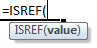(Notice how the formula inputs appear)

### ISREF Function Syntax and Inputs:

value – The test value

## How to use the ISREF Function

The ISREF Function tests if an input is a valid cell reference or not.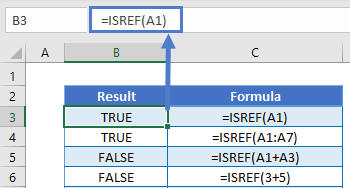Here, A1 and A1:A7 are valid cell references so the ISREF Function returns TRUE for those inputs.

### Deleted References

Let’s delete column A from the above example. After deleting column A, the ISREF Function returns FALSE for references to former column A.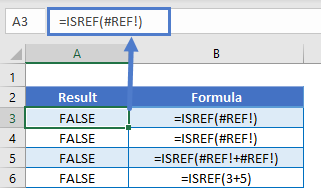You can see that one of the primary uses of the ISREF Function is to test if a range still exists or if it had been deleted.

### Does Worksheet Exist

Another possible usage of the ISREF Function is to test if a worksheet exists. In this example, we will enter a cell reference to another worksheet =Sheet2!A2 .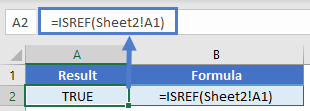Because the worksheet exists, the cell reference is valid and TRUE is returned.

Now let’s delete Sheet2 and you can see that the value is now FALSE.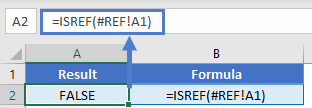### INDIRECT and OFFSET

Excel provides you with two functions to dynamically create ranges: INDIRECT and OFFSET. By using these functions with ISREF, you can test if a range is valid.

Let’s look at an INDIRECT example to test if a worksheet exists by referring to the worksheet in a cell.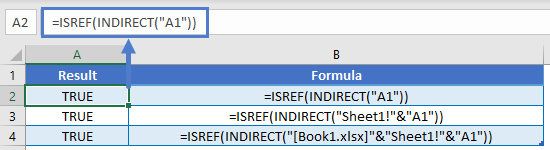Now you can change the worksheet input to test if various sheets exist.

Next, let’s look at an OFFSET example. Here we will test if the range resulting from the OFFSET Function is valid.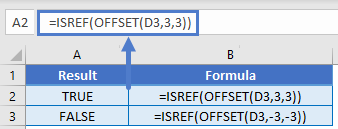The second example, returns an invalid range (Row < 1), so ISREF returns FALSE.

You might use these function combinations along with an IF Statement like this: